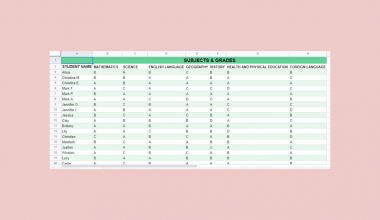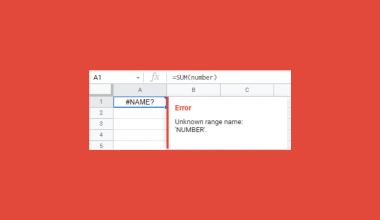# How to Use CONVERT Function in Google Sheets

The CONVERT function in Google Sheets is useful if you want to convert a number in one measurement system to another.

Meaning, the CONVERT function returns the equivalent unit measurement of a given value to a different measurement system.

The rules for using the CONVERT function in Google Sheets are as follows:

• The units and prefixes passed to the CONVERT function are case-sensitive.
• The CONVERT function will return the #N/A error when the units and prefixes are not recognized and compatible.
• The CONVERT function will return the #VALUE! error when the provided value is not valid.
• The CONVERT functions supports the conversion of units in weight, distance, time, pressure, force, energy, power, magnetism, temperature, liquid, volume, area, information, metric prefixes, and, binary prefixes.

Let’s take an example.

Adriana, a stock clerk, is trying to come up with the list of ribbons currently in their inventory together with the available colors and their measurement in meters.

However, her workmate seemed to have listed the measurement in yards.Now, she has to find a way to convert them into meters, without having to worry about the formula to use.

Luckily, a friend taught her a few tricks in utilizing Google Sheets on her day-to-day routine in the store.

With the help of the CONVERT function, Adriana was able to convert the length of each ribbon type from yards to meters.She should now be able to identify which type of ribbon needs re-stocking.

Watch out for a more advanced tutorial and examples on how you can use the CONVERT function in the coming weeks. Be sure to subscribe to be notified.

Perfect! Let’s begin getting to know more about our CONVERT function in Google Sheets.

## The Anatomy of the CONVERT Function

So the syntax (the way we write) the CONVERT function is as follows:

```=CONVERT(number,from_unit,to_unit)
```

Let’s dissect this thing and understand what each of these terms means:

• = the equal sign is just how we start any function in Google Sheets. It is how Google Sheets understand that we are asking it to either do computation or use a function.
• CONVERT() this is our CONVERT function. It converts a value from one unit of measurement to another unit of measurement.
• number is the value to be converted.
• from_unit is the starting unit for the number. It needs to be enclosed with quotation marks.
• to_unit is the ending unit for the result.  It needs to be enclosed with quotation marks.

## A Real Example of Using CONVERT Function

Let’s take a look at the inventory that Adriana prepared below to see how the CONVERT function is used in Google Sheets.In the first type of ribbon above, Adriana converts the given length in yards into meters by providing 3 arguments to the CONVERT function.

=CONVERT(B2,”yd”,”m”)

The first argument is cell B2, where the measurement in yards is located.

The second argument identifies what unit of measurement the value in B2 is. In this case, it’s “yd”, which for the CONVERT function means the “yard” unit.

Lastly, the third argument is the value “m”, which is for the CONVERT function to convert the value in the first argument into meters.

This means that the CONVERT function will convert the unit of measurement of the value in B2 from yards into meters.The same logic applies to the CONVERT function used to convert the measurement of the remaining ribbon colors.How hard can that be?

Well, worry no more. All she has to do is edit the formula and change the third argument from “m” to “cm”.The CONVERT function accepts certain text values for from_unit and to_unit. For the list of some acceptable text values, feel free to make a copy of the spreadsheet using the link I have attached below.

## How to Use CONVERT Function in Google Sheets

1. Click on any cell to make it the active cell. For this guide, I will be selecting C2, where I want to show the result.1. Next, type the equal sign ‘=‘ to begin the function and then follow it with the name of the function, which is our ‘convert‘ (or ‘CONVERT‘, not case sensitive like our other functions).1. Type open parenthesis ‘(‘ or simply hit Tab key to let you use that function.1. Now the exciting part! Let’s give our function its first argument, the number.  You may pass constant data by typing the exact value after the parenthesis. Just don’t forget to enclose it with quotation marks (“”). In this case, type in ‘133’.1. To let the Google sheet know that we’re done typing our first argument, we should now type in the delimiter or the character that separates each argument on a function. In this case, type comma,’.1. Type in our second argument, which is the from_unit. Type in the text string ‘yd‘. Just be sure to enclose it with quotation marks (“”). Alternatively, you may use a cell address that contains the unit ‘yd’. In this case, you can type in ‘yd’. Take note that you have to enclose it with quotation marks.1. End your second argument by typing in another comma (‘,). Now, pass the third argument, which is the to_unit. Like the second argument, you can either pass a direct unit, in which case you need to enclose it with quotation marks (“”), or a cell address containing the unit. In this case, type in ‘m and enclose it with quotation marks.1. Finally, hit your Enter or Tab key. Cell C2 will now show you the return value of the CONVERT function, or the measurement in meters.1. Notice that in this example, we provided the exact date value, constant, as the first argument of our CONVERT function. Alternatively, this constant can be a variable or simply a cell address containing your value.

1. Edit the formula in cell C2 by changing the number into the cell address. In this case, type in ‘B2’.1. Hit your Enter or Tab key. Cell C2 will now show you the same result.1. Copy the formula down to cells C3 to C8.That’s pretty much it. You can now use the CONVERT function in Google Sheets together with the other numerous Google Sheets formulas to create even more powerful formulas that can make your life much easier.Our goal this year is to create lots of rich, bite-sized tutorials for Google Sheets users like you. If you liked this one, you'll love what we are working on! Readers receive ✨ early access ✨ to new content.

##### You May Also Like## How to Use FVSCHEDULE Function in Google Sheets

The FVSCHEDULE function in Google Sheets is useful when you want to know the future value of an…## How to Color Alternate Rows in Google Sheets

To color alternate rows in Google Sheets is useful if you have a large set of data, with…## How to Fix ‘Formula Parse’ Error in Google Sheets

A formula parsing error message appears when the formula inputted expects a specific data type, but it received…

## How To Use ISNONTEXT Function in Google Sheets

The ISNONTEXT function in Google Sheets is used when you need to determine whether the value of a…